Disclosure: This post may contain affiliate links, meaning when you click the links and make a purchase, we receive a commission.

# Flat and reducing rate of interest calculator in Excel [Free Download]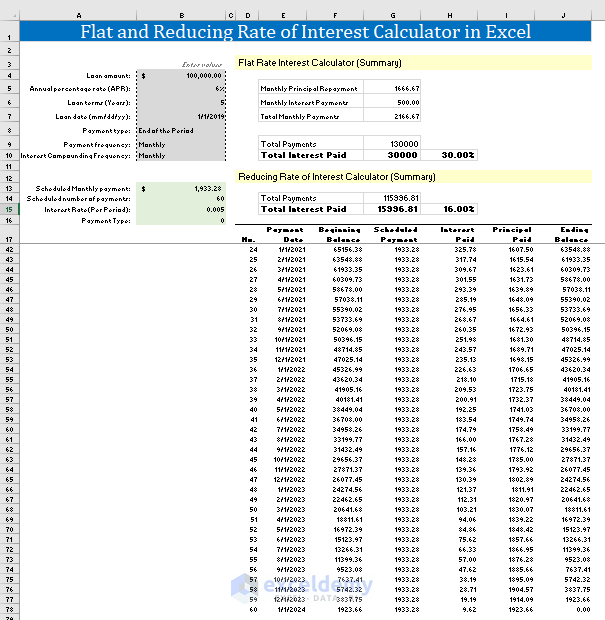Flat and reducing rate of interest calculator in Excel

“No Macro used. No installation is necessary. Just download and start using.” – Kawser Ahmed (Calculator Developer)

Created using Excel 2016 version

License: Personal Use (Not for resale or distribution)

Let us know (in the comment box) your criteria or the problems that you’re facing while using this template. We shall update the template.

Many people fail to understand these two financial terms: Flat Rate Interest and Reducing Balance Rate.

When you’re dealing with your personal finance, it is not wise to be in dark. Not knowing general financial concepts can lead you to financial disasters. If you read this article, you will be clear about these two financial terms.

I will also provide you the Flat and Reducing rate of interest calculator in Excel. Using the calculator, you will be able to see which methods give you a better financial return.

## What is Flat Rate Interest?

Flat Rate Interest = (Loan Amount x Number of Years x Annual Percentage Rate) / Total Number of Installments

It is better to understand this definition with an example.

Suppose, you took a loan of amount \$100,000 with an annual percentage rate (APR) 6%. You took the loan for 5 years and you have to pay the installment every month.

Your Flat Rate Interest will be:

(\$100,000 x 5 x 6%) / 60

= \$30,000 / 60

= \$500

This is your interest that you will pay in every installment.

Now let’s calculate your principal repayments.

Principal Repayment = Loan Amount / Total Number of Installments

= \$100,000 / 60

= \$1,666.67

So, your total monthly payment will be: \$500 + \$1666.67 = \$2166.67

You see the summary of the above calculation (using my Flat Rate Interest Calculator) in the following image. The calculator also shows the following things:

• Total Payments: \$130,000
• Total Interest Paid: \$30,000
• You will pay an overall 30% interest rate for the next 5 years. The rate is high, right?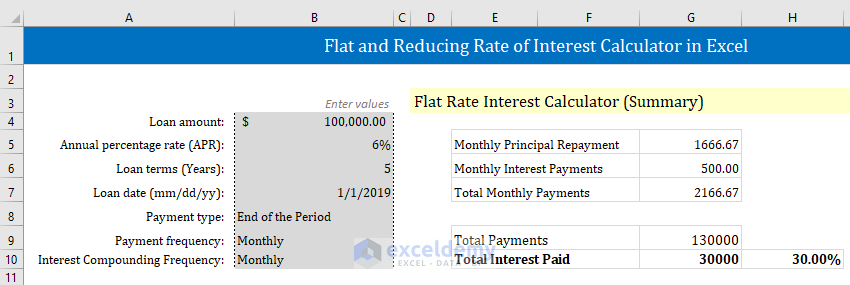## What is Reducing the Rate of Interest?

This is a better approach when you will handle your loan.

And this is widely used by banks and financial institutions.

We will calculate the Reducing Rate of Interest using the above loan details.

• A loan of amount \$100,000
• Annual percentage rate (APR) 6%
• Tenure of the Loan: 5 years
• Payment Frequency: Monthly

If the Payment Frequency is Monthly, then at first, we have to calculate the Rate for a month:

= Annual Percentage Rate / 12

= 6% / 12

= 0.5%

= 0.005

Next thing is: we have to calculate monthly PMT for the loan using Excel’s PMT function:

=PMT(rate, nper, -loan)

Here,

• rate = 0.005
• nper = 60; [nper = number of total periods]
• -loan = -100,000; [loan is negative as we want the PMT as a positive value]

It returns the value: \$1933.28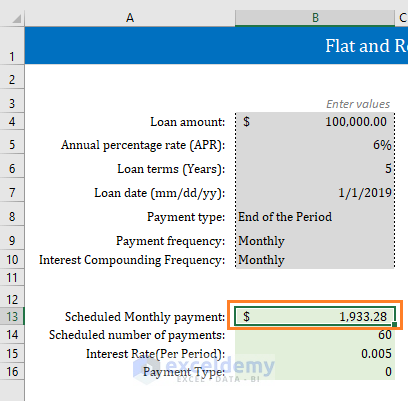Now let’s discuss how the whole thing works:

1st Month:

At the start of the first month, you just took the loan. So, the balance \$100,000.

At the end of the first month, you’re paying \$1933.28 (PMT amount) to the financial intuition.

How much of this amount (\$1933.28) is paid to interest?

Your first month interest is: \$100,000 x 0.005 = \$500.

So, \$500 of the amount of \$1933.28 is provided as the interest payment.

The rest, \$1933.28 – \$500 = \$1433.28, will be deducted from your principal.

So, at the end of the first month, your new principal will be: \$100,000 – \$1433.28 = \$98,566.72

2nd Month:

At the start of the 2nd month, your new principal is: \$98,566.72

At the end of the second month, you’re paying \$1933.28 (PMT amount) to the financial intuition.

How much of this amount (\$1933.28) is paid to interest?

Your second month interest is: \$98566.72 x 0.005 = \$492.83.

So, \$492.83 of the amount of \$1933.28 is provided as the interest payment.

The rest, \$1933.28 – \$492.83 = \$1440.45, will be deducted from your principal.

So, at the end of the second month, your new principal will be: \$98566.72 – \$1440.45 = \$97126.27

This is how the whole process advances.

In the following images, you show the whole process.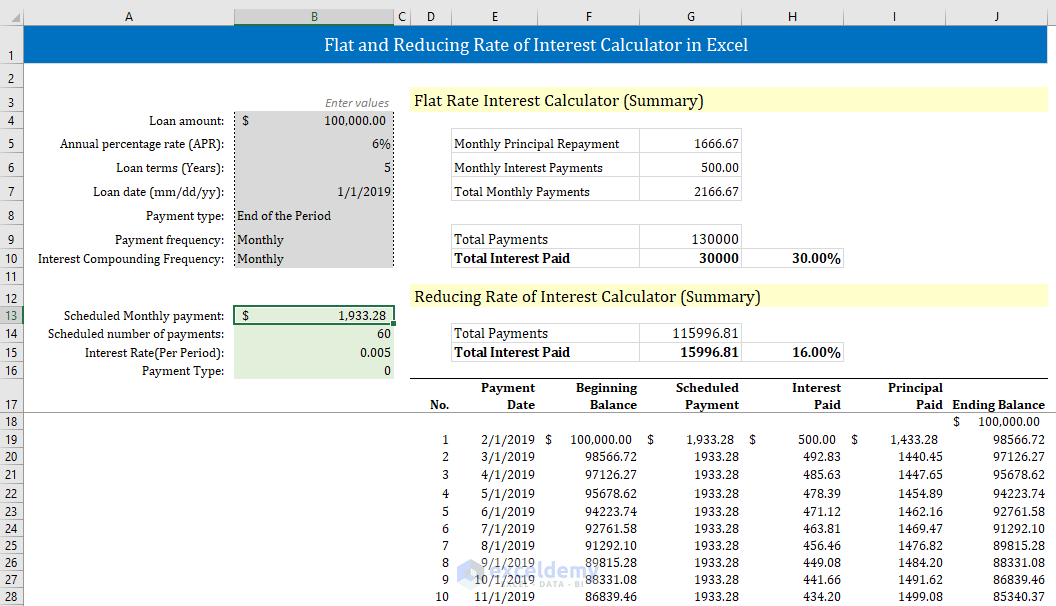You see from the following image that at the last payment, we pay only 9.62\$ as the interest payment and we clear all our principal with the rest amount \$1923.66. Our ending balance is 0.00. So that is our last payment to the financial institution.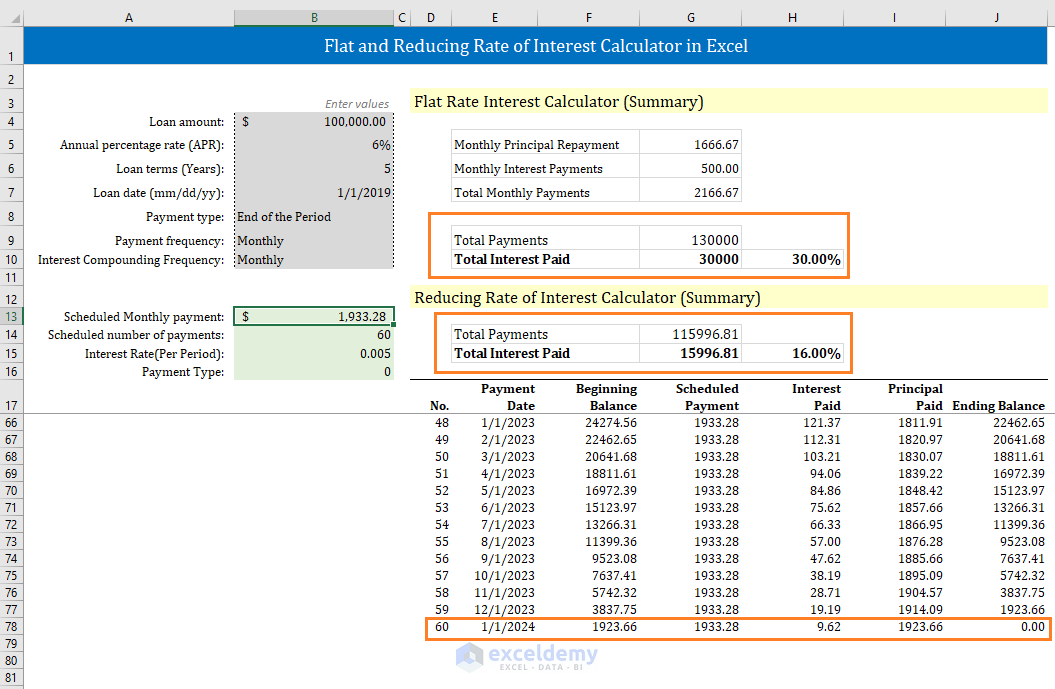You see the summary of the loan using the Reducing Rate of Interest.

At the end of the day, we only pay 16% interest overall in this system.

## How will you use this calculator?

It is very easy to use this calculator.

Enter the following values to use this calculator:

• Loan Amount
• Annual Percentage Rate (APR)
• Loan terms (Years)
• Loan Date (mm/dd/yy)
• Payment type. It is a drop-down list. You will get two types of payments. End of the Period and Beginning of the Period. Choose one that suits your loan
• Payment frequency: It is also a drop-down list. You can choose payment frequencies:
 Interest Compounded Calculated After (Days or Months) No. of Payments/Year Weekly 7 Days 52 Bi-weekly 14 Days 26 Semi-monthly 15 Days 24 Monthly 1 Month 12 Bi-monthly 2 Months 6 Quarterly 3 Months 4 Semi-annually 6 Months 2 Yearly 12 Months 1
• Interest Compounding Frequency: In most cases, your Payment frequency will be equal to Interest Compounding Frequency. In some countries, for example, Canada, Interest is compounded semi-annually but payment is done monthly. So, except in rare cases, your Payment frequency will be always equal to Interest Compounding Frequency.

There are no other values to input. The rest will be handled by the Calculator.

## Conclusion

So, it is clear that you will pay less interest in the Reducing Rate of Interest than the Flat Interest Rate. Always choose the method that costs you less. If you face any difficulties to use this calculator, let us know in the comment box.

Hello!
Welcome to my Excel blog! It took me some time to be a fan of Excel. But now I am a die-hard fan of MS Excel. I learn new ways of doing things with Excel and share here. Not only how to guide on Excel, but you will get also topics on Finance, Statistics, Data Analysis, and BI. Stay tuned!

1. Replylisa Apr 12, 2019 at 12:51 PM

such a great help in my work since i handled loan applications. how about the pro-rata interest ? example the loan is granted on the 10th of the month. how do i include in the calculator the pro-rata interest. the payment is always at the end of the month so how do i include the pro-rata days to include in the monthly payment

2. Replyravneet Aug 29, 2019 at 3:42 PM

hello myself ravneet from patiala i want to know which is best for small finance bank (jivan etc)
1st and last installment change other remain same send formala detail for excel to find out

3. ReplyDec 21, 2019 at 5:23 PM

Hi,
Have gone through the template and found it quite interesting and will score it 8 out 10 reason:
1. On Web most of the experts had shared template and urs is having same feasible solution. But have given two addition points in 8 belongs to combine approach of Straight line and reducing Method.

2. ‘ve hold 2 points for one unique reason, suppose Mr. X had taken loan of USD 150000 for 5 Years at 18% intt. rate and at the end of 23rd installment he paid USD 60000 than in that particular scenario template got failed.

Hoping that you got my point.

4. ReplyEdward Walker Feb 20, 2020 at 9:49 PM

Hi, I m trying to learn how to use excel sheets. The one thing I really need but have not figured out is this.

I want to be able to put in a fixed value, that is changed with a set interest rate monthly, and able to auto calculate the new balance over a set number of months. The interest rate needs to be where it can be either a positive or negative value. could you share a formula that excel could use for this purpose?

5. ReplyGeorge Arce, Jr. Jun 10, 2020 at 10:07 AM

What if I have a fixed payment schedule? How do I handle interest calculations around that?